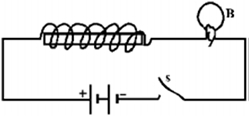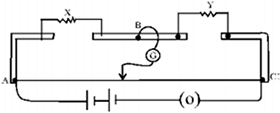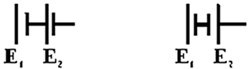# What is the De Broglie wavelength associated with an electron moving with a speed of 5.4×106m/s, and a ball of mass 150g travelling at 30.0 m/s? The half-life of92U238undergoing α- decay is 4.5×109years. What is the activity of 1g sample of92U238? A regular hexagon of side 10 cm has a charge 5µc at each of its vertices. Calculate the potential at the centre of the hexagon. Double convex lenses are to be manufactured from a glass of refractive index 1.55 with both faces of the same radius of curvature. What is the radius of curvature required if the focal length is to be 20 cm? Three capacitors of capacitance 2pF, 3pF and 4pF are connected in parallel. What is the total capacitance of the combination? Determine the charge on each capacitor if the combination is connected to a 100V supply. What is the focal length of a convex lens of focal length 30 cm in contact with a concave lens of focal length 20 cm? Is the system a converging or a diverging lens? Fig. shows a light bulb (B) and iron cored inductor connected to a DC battery through a switch (S). What will one observe when switch (S) is closed? How will the glow of the bulb change when the battery is replaced by an ac source of rms voltage equal to the voltage of DC battery? Justify your answer in each case.The given figure shows the experimental set up of a metre bridge. The null point is found to be 60cm away from the end A with X and Y in position as shown.When a resistance of 15Ω is connected in series with ‘Y’, the null point is found to shift by 10cm towards the end A of the wire. Find the position of null point if a resistance of 30Ω were connected in parallel with ‘Y’. Why is a potentiometer preferred over a voltmeter for determining the emf of a cell? Two cells of emf E1and E2are connected together in two ways shown here.The 'balance points' in a given potentiometer experiment for these two combinations of cells are found to be at 351.0cm and 70.2cm respectively. Calculate the ratio of the emfs of the two cells.

Dear Student,
41.

kindly post different question in different threads
Regards

• -1
De Broglie Wavelength formula:-
λ = h/mv, where λ is wavelength, h is Planck's constant, m is the mass of a particle, moving at a velocity v